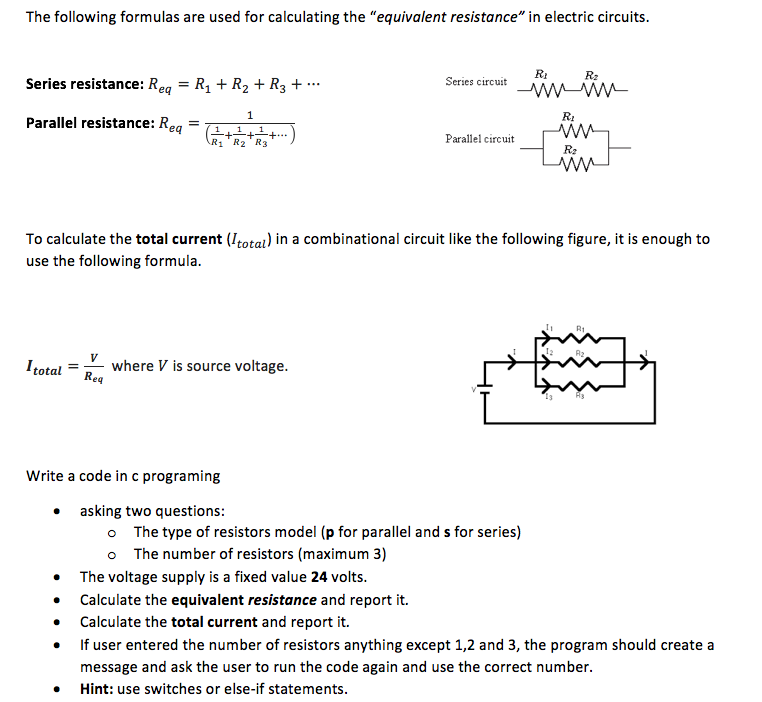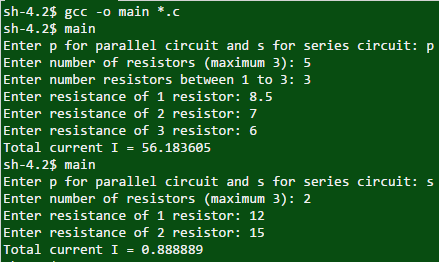# Question & Answer: The following formulas are used for calculating the "equivalent resistance" in electric circuits. Series resistance:R_eq…..The following formulas are used for calculating the “equivalent resistance” in electric circuits. Series resistance:R_eq = R_1 + R_2 + R_3 +. .. Parallel resistance:R_eq = 1/(1/R_1 + 1/R_2 + 1/R_3 +. . .) To calculate the total current (I_total) in a combinational circuit like the following figure, it is enough to use the following formula. I_total = V/R_eq where V is source voltage. Write a code in c programing asking two questions: The type of resistors model (p for parallel and s for series) The number of resistors (maximum 3) The voltage supply is a fixed value 24 volts. Calculate the equivalent resistance and report it. Calculate the total current and report it. If user entered the number of resistors anything except 1, 2 and 3, the program should create a message and ask the user to run the code again and use the correct number.

Program :

Program :

Don't use plagiarized sources. Get Your Custom Essay on
Question & Answer: The following formulas are used for calculating the "equivalent resistance" in electric circuits. Series resistance:R_eq…..
GET AN ESSAY WRITTEN FOR YOU FROM AS LOW AS \$13/PAGE

#include <stdio.h>
int main()
{
float resistance,eq_resistance=0,I;   // variable declaration
int v=24,number,i; // variable declaration
char ch,ch1;       // variable declaration
printf(“Enter p for parallel circuit and s for series circuit: “);
scanf(“%c”,&ch);           // accept the choice
printf(“Enter number of resistors (maximum 3): “);
scanf(“%d”,&number);               // Accept number of resistors
while(number>3||number<1)     // check number of resistors between 1 to 3
{
printf(“Enter number resistors between 1 to 3: “);
scanf(“%d”,&number);
}
if(ch==’p’)              // if choice is parallel circuit
{
for(i=1;i<=number;i++)   // loop runs from 1 to the number
{
printf(“Enter resistance of %d resistor: “,i);
scanf(“%f”,&resistance);          // accept the resistance
eq_resistance+=1/resistance; // calculate equivalent resistance
}
}
else
if(ch==’s’)
{
for(i=1;i<=number;i++)
{
printf(“Enter resistance of %d resistor: “,i);
scanf(“%f”,&resistance);          // accept resistance
eq_resistance+=resistance;    // calculate equivalent resistance
}
}
I=v/eq_resistance;                       // calculate the current
printf(“Total current I = %fn”,I);
return 0;
}

Output :